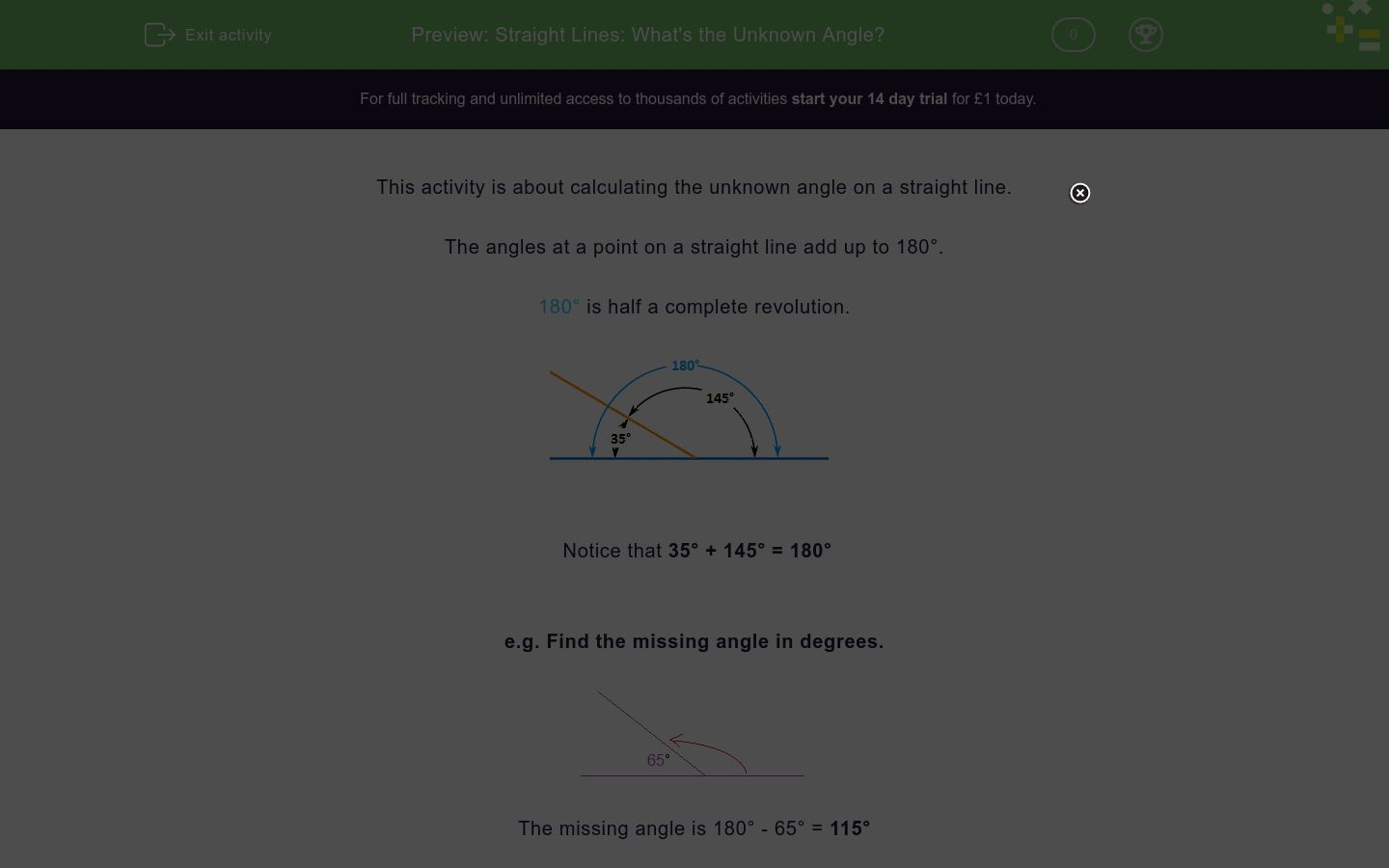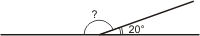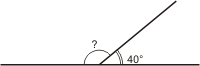# Straight Lines: What's the Unknown Angle?

In this worksheet, students calculate the unknown angle on a straight line.Key stage:  KS 2

Curriculum topic:   Geometry: Properties of Shapes

Curriculum subtopic:   Identify Angles at a Point

Difficulty level:### QUESTION 1 of 10

This activity is about calculating the unknown angle on a straight line.

The angles at a point on a straight line add up to 180°.

180° is half a complete revolution.Notice that 35° + 145° = 180°

e.g. Find the missing angle in degrees.The missing angle is 180° - 65° = 115°

Want to understand this further and learn how this links to other topics in maths?

Why not watch this video?

Calculate the unknown angle in degrees.  (Just write the number)Calculate the unknown angle in degrees.  (Just write the number)Calculate the unknown angle in degrees.  (Just write the number)Calculate the unknown angle in degrees.  (Just write the number)Calculate the unknown angle in degrees.  (Just write the number)• Question 1

Calculate the unknown angle in degrees.  (Just write the number)160
EDDIE SAYS
180 - 20 = 160
• Question 2

Calculate the unknown angle in degrees.  (Just write the number)40
EDDIE SAYS
180 - 140 = 40
• Question 3

Calculate the unknown angle in degrees.  (Just write the number)60
EDDIE SAYS
180 - 120 = 60
• Question 4

Calculate the unknown angle in degrees.  (Just write the number)140
EDDIE SAYS
180 - 40 = 140
• Question 5

Calculate the unknown angle in degrees.  (Just write the number)120
EDDIE SAYS
180 - 60 = 120
---- OR ----

Sign up for a £1 trial so you can track and measure your child's progress on this activity.

### What is EdPlace?

We're your National Curriculum aligned online education content provider helping each child succeed in English, maths and science from year 1 to GCSE. With an EdPlace account you’ll be able to track and measure progress, helping each child achieve their best. We build confidence and attainment by personalising each child’s learning at a level that suits them.

Get started